## Table of ContentOpen Access

ARTICLE

# ACO-Inspired Load Balancing Strategy for Cloud-Based Data Centre with Predictive Machine Learning Approach

Niladri Dey1, T. Gunasekhar1, K. Purnachand2,*

1 Department of CSE, K. L. University, Vaddeswaraam, 522302, Andhra Pradesh, India
2 Department of CSE (Data Science), B. V. Raju Institute of Technology, TS, 502313, India

* Corresponding Author: K. Purnachand. Email:Computers, Materials & Continua 2023, 75(1), 513-529. https://doi.org/10.32604/cmc.2023.035139

## Abstract

Virtual Machines are the core of cloud computing and are utilized to get the benefits of cloud computing. Other essential features include portability, recovery after failure, and, most importantly, creating the core mechanism for load balancing. Several study results have been reported in enhancing load-balancing systems employing stochastic or biogenetic optimization methods. It examines the underlying issues with load balancing and the limitations of present load balance genetic optimization approaches. They are criticized for using higher-order probability distributions, more complicated solution search spaces, and adding factors to improve decision-making skills. Thus, this paper explores the possibility of summarizing load characteristics. Second, this study offers an improved prediction technique for pheromone level prediction over other typical genetic optimization methods during load balancing. It also uses web-based third-party cloud service providers to test and validate the principles provided in this study. It also reduces VM migrations, time complexity, and service level agreements compared to other parallel standard approaches.

## Keywords

1  Introduction

Barzoki et al.  found a growing need for scalable, high-performance applications. Akbari et al. suggested researching biology-based algorithms . Current research focuses on making algorithms to divide work without losing virtualization’s benefits. This chapter demonstrates a natural way to balance loads. Next, the paper explains why more research is needed. Henceforth, it is natural to realize the demand for further optimizations. These algorithms are primarily driven by the rule engine and cannot encourage dynamic rule building, making them less dynamic and responsive to load balancing. These strategies are also less effective in terms of proper virtual machine utilization. Finally, it is discovered that these mechanisms are bottlenecked for reducing response time beyond a certain scale. Thus, this paper briefly aims to evaluate the possibility of summarizing load characteristics. This study also provides a better prediction technique for pheromone level prediction than other common genetic optimization methods used during load balancing. However, finding the best solution usually depends on how the problem is set up and, to a large extent, on the state of the data fed into the algorithms. So, optimization techniques are often used to make further improvements and find the best solution to a problem.

Further, this paper is organized such that, in Section–2, the foundational understanding of this domain of the research is furnished, the baseline methods for the load balancing are discussed to evaluate research gaps, in Section–3 as proposed algorithms, further this paper furnishes the obtained results and discuss the improvements compared with the parallel other research outcomes in Sections–4 and 5, the research conclusion is furnished.

2  Background of the Research

In this Section of the work, the foundation layout of the research is furnished. Bio-Inspired methods are widely popular in various fields of research. Every research in various fields proposes multiple algorithms to solve domain-specific problems, and the solutions by these algorithms lead to specific problem solutions. Nevertheless, algorithms often research to some extent where further improvements cannot be proposed, only relying on the core strategical solutions, and many of these algorithms provide multiple solutions, which are all timely and effective. However, finding the most optimal solution habitually relies on the problem environment, which can be significantly represented by the state of the data as input to the algorithms. Thus, optimization techniques are widely used for further improvements and to find the optimal solution for the problem. Apart from finding the best solution or the solution space, the optimization algorithms on top of the primary problem-solving algorithms can cater to a wide range of solutions. The Bio-Inspired optimization algorithms can be primarily categorized into two different categories:

2.1 Deterministic Methods

The first category is the deterministic methods. The deterministic method of the problem solution ensures the optimization solution in the complete solution space. The deterministic algorithms usually consider the features or the parameters which describe the problem in detail and thus guarantee to find the global solution to the same problem. Nevertheless, the deterministic methods are limited to solving the problem with less or no information from the outside and are generally treated as black box problems. Also, this method is difficult to solve in the case of the problem, which is highly unpredictable and frequently changes the pattern of the transitions. Further, these methods can also be difficult to apply to some problems, which deal with a higher order of the data and comparatively large dependencies, as demonstrated in work by Li et al. . There are many prominent methods in this optimization category, such as Branch & Bound, Cutting Plane, Inner & Outer approximation, and convex methods.

2.2 Stochastic Method

The second category of them is the stochastic methods. These methods can find the solution in finite time and faster than the deterministic methods. Nonetheless, the stochastic methods cannot provide the optimal global solution for any problem solution space. The stochastic methods can only find the probabilistic solution in finite time, as showcased and proven by Zhou et al. . The wide acceptance of the stochastic methods is driven by various advantages such as:

Firstly, less complex mathematical modeling can still be useful for finding the solution. Secondly, the case of large-scale data-driven problems, the recent problem trend, can be well handled by the stochastic methods as elaborated in work by Yan et al. .

Lastly, the time to find the optimal solution, the optional probabilistic solution, is less than the deterministic methods. The popular optimization algorithms under the stochastic methods are genetic algorithms, ant colonies, artificial bee colonies, particle swarms, and many more. This work also proposed a novel optimization method using bio-inspired strategies on the edge of the stochastic optimization method. Further, this work elaborates on the possibilities of applying bio-inspired optimization methods to solve cloud computing load-balancing problems. Nevertheless, these algorithms are criticized for the following reasons:

•   These algorithms are primarily driven by the rule engine. They cannot encourage dynamic rule building, which makes these algorithms less dynamic and less responsive to load balancing.

•   These strategies are also less effective in properly utilizing virtual machines.

•   Finally, these mechanisms are found to be bottlenecked for reducing the response time beyond a certain scale.

Thus, these problems demand further research. In this chapter of the work, the problems encountered by the parallel research attempts are addressed with a novel bio-inspired mechanism for optimizations. The next Section of the work addresses the fundamental strategy for load balancing on cloud computing, and the principle mathematical model is analyzed.

3  Formulation of the Research Problem and Proposed Load Summarization Process

This portion of the paper discusses the basic technique for load balancing and summary. The underlying idea of load balancing is virtual machine migration. Thus, the load computation and migration methods must be well understood. According to Medina et al. , the commonly acknowledged procedure of migrating virtual machines from the source physical resource pool to the destination physical resource pool is termed live migration. The source virtual machines and services are not stopped, and the destination virtual machines and services are not restarted, but all maintenance activities may be done using kernel procedures. This technique improves availability and service level violations compared to other parallel solutions.

3.1 Formulation of the Research Problem

The flaws in the current methods have been exposed by the discovery of the fundamentals of load succinct summation, primitive optimization methods, and recent research on the primitive method. From now on, the issues that need to be addressed in this study will be outlined in this Section of the paper.

During this phase, two problems are recognized and formulated so that the solutions can be modeled in greater detail.

Primitive and enhanced ACO methods have both been criticized for being overly complex when applied to large search spaces, as discussed in the previous part of this document. Thus, to demonstrate the reduction potential of predictive methods, the following equation lemma is formulated:

Lemma–1: The prediction of the future load conditions shall lead to a significant reduction of the time complexity for any load-balancing algorithm.

Proof: The standard analogy compares the performance or the time complexity for two load balancing strategies, wherein in the first instance, the Algorithm calculates the load situations reactively, and in the second instance, the Algorithm calculates the load conditions proactively or using predictive analysis. However, the standard load analysis algorithms are furnished into four primary phases identification of the load condition (LC), identification of the instance capacity (IC), load optimization (LO), and finally, migration between the instances (MB). Here for reactive algorithms, assuming that these four phases take the time as t1, t2, t3, and t4, respectively with the total time as T. Thus, this can be realized as,

T=t1+t2+t3+t4(1)

Naturally, the first phase of identification of the load condition is highly repetitive and must be added as a service protocol to the data center architecture. Hence the time t1 is significantly higher compared to the other three phases’ time complexity. As

t1>t2|t3|t4(2)

Similarly, it is natural to realize that the optimization phase is also iterative and must be almost equal to the identification phase. Also, the migration phase may be flexible, but due to the larger size of the virtual machine, may take longer. Hence, the complete comparison between the time complexities in various phases can be identified as,

t1>t4>t3>t2(3)

Alternatively, during the proactive or the predictive strategy for the same Algorithm, assuming that the standard load analysis algorithms are furnished into four primary phases identification of the load condition (LC), identification of the instance capacity (IC), load optimization (LO) and finally migration between the instances (MB). Here for reactive algorithms, assuming that these four phases take the time as t11, t22, t33, and t44, respectively with the total time as T1. Thus, this can be realized as

T1=t11+t22+t33+t44(4)

Fundamentally, for the predictive strategy-driven algorithms, the iterative phases must be converted into a single-step process and can be computed during the previous step of the algorithms. As the identification of the load condition must be completed during the migration process of the previous phase. The separate time taken for identifying the load condition tasks is completed within the same time limit of the migration process. As

t11t44(5)

Henceforth, this understanding must also be incorporated with the previous assumptions, as stated in Eq. (2),

T1=t11|t44+t22+t33(6)

These enhancements to the Eq. (6) can be justified with the following relation,

Ift11t44,thenT1=t11+t22+t33Else,thentimeT1=t44+t22+t33(7)

Hence,

T>>T1(8)

Consequently, this mathematical formulation proves that load prediction can substantially decrease the computational complexity of the load-balancing Algorithm using predictive calculations.

Secondly, as discussed in the previous sections of this work, the ABC or BAT methods, both primitive and enhanced methods, are criticized for less stability due to incorporating the various parameters. Henceforth, in the following mathematical model using the correlation method, the stability of the proposed model is aimed to be increased.

Lemma–2: The correlation-based attribute reduction reduces the time complexity and model stability to a greater extent.

Proof: In this mathematical formulation, the non-correlation-based strategy is compared with the correlation-based strategy. Assuming that the non-correlation-based model is deployed with a set of n parameters as P [], and each parameter in the model is denoted as pi, then this relation can be formulated as,

P[]=i=1npi(9)

Also, if processing each parameter for the fitness function is t time, then for total time, T1, for processing the fitness function can be formulated as,

T1=nt(10)

On the other hand, if n number of parameters can be reduced to m number of parameters using the correlation factors, then a set of m parameters as P1[] and each parameter in the model is denoted as p1i, can be formulated as,

P1[]=i=1mp1i(11)

where the n number of parameters can be converted into a lesser number of parameters, m, using the correlation method with X as correlation factors, then each parameter in the reduced set can be formulated as,

p1i=β1.pi+β2.pi+1+β3.pi+2+..(12)

Again, if processing each parameter for the fitness function is t time, then for total time, T2, for processing the fitness function can be formulated as,

T2=mt(13)

Here it is significant to realize that m<<n the following relation can be easily derived,

T1>>T2(14)

Thus, with this mathematical formulation, it is conclusive that the correlation-based attribute reduction reduces the time complexity and model stability to a greater extent. The proposed predictive model is presented in the next Section of this work. Henceforth, based on the problem identifications and mathematical problem modeling, the proposed solutions with the mathematical models are furnished in the next Section of this work.

3.2 Mathematical Model for the Proposed Solutions

After understanding the load summarization and balancing process, primitive and recent methods for optimizations with the fundamental bottlenecks, and formulating the problems to be solved, the mathematical models for the proposed solutions are furnished in this Section of the work. This Section of the work discusses 2 major solutions, apart from the contribution of load summarization in the previous Section for predictive analysis of the load balancing mechanism with the correlation-based fitness function minimizations. Firstly, as demonstrated in Lemma–2, the incorporation of the predictive model for pheromone level is formulated.

Lemma–3: The corrective coefficient-based pheromone prediction analogy can improve the performance of the load-balancing strategy.

Proof: The corrective coefficient-based pheromone prediction strategy is formulated in this mathematical formulation. Assuming that the following is the network physical resource pool availability. In this model example, there are a total of 4 physical resource pools configured as N1, N2, N3, and N4. Here N1 physical resource pool is categorized as the source or the overloaded physical resource pool, and other nodes are identified destinations or available physical resource pools for migration from the source. As this optimization process can be deployed seamlessly and the existing strategy is assumed to be already deployed with the ACO method, thus there are some initial pheromone levels exists on each path as traces of the previous virtual machine migrations. Thus, some pheromones exist for every path as PH1 to PH6, respectively. Also, assuming that any given virtual machine, Vi, is allocated the physical resource pools as n number of computes, C, m number of memory units, M, k number of storage units, S and finally, p number of network resources, N. Thus, the load, L, at a specified time point, t, can be presented as,

L(t)=x=1nCx+x=1mMx+x=1kSx+x=1pNx(15)

Further, if the pheromone level at a given time t can be represented as PH(t) and at time t+1, the pheromone level can be presented as PH(t+1). The increase or the decrease of the pheromone level can be formulated as,

PH(t+1)=PH(t)±Δτ(16)

The factor Δτ defines the deposition of evaporation of the pheromone as the positive sign denotes the deposition and the negative sign denotes the evaporation. Here, this proposed method deploys the predictive analogy for pheromone level prediction as,

PH(t+1)=PH(t).e±KT(17)

The e±KT defines the decay or growth in the rate of pheromone deposition or evaporation, where ±K defines the rate of growth or decay, which is again controlled by the sign as positive or negative and T denotes the life span of the path or network connection span in this case. Also, the growth or decay rate is a time-dependent variable, which must be decided using the trend, TR(t), and the prediction depth, DP(t). Regardless to mention, these two new variables are also time-dependent. Hence, the growth of the decay rate can be formulated as,

K(t)=TR(t)+DP(t)(18)

where the greater number of increases or decay in the pheromone levels can decide the trend,

TR(t)=K+veKKveK(19)

Furthermore, the depth of the prediction is the number of historical data points considered. Regardless, as this proposed method relies on the pheromone level prediction, thus the correction during the prediction phases is highly important. Hence, this method deploys the correction strategy with the help of the mean square error α as the correction coefficient method as formulated here,

α=i=1n|ki(t)2ki(t)2|n(20)

Here, ki(t) it defines the actual growth or decay and ki(t) the predicted growth or decay for n number of notes at time t. Finally, the prediction of the pheromone level for any given path can be formulated with the help of Eqs. (17)(20) as,

PH(t+1)=PH(t).e±K+veK(t)KveK(t)+DP(t).T±α(21)

The proof of the performance improvement is already established in the previous Section of this work. As demonstrated in Lemmas–2 and 3, incorporating correlation-based parametric reduction shall reduce the complexity.

Lemma–4: Correlation-based parameter reduction can improve the performance of the load-balancing strategy.

Proof: The correlation-based parameter-reduction strategy is formulated in this mathematical formulation. In this example, we assume that each virtual machine (Vi) has a variable number of processors, C, m multitude of memory units, M, and ultimately p number of social reserves, N, in its physical resource pool. In this way, the load at a given time, t, can be expressed as L,

L(t)=x=1nCx+x=1mMx+x=1kSx+x=1pNx(22)

Also, assuming that any given destination resource pool, Di, is allocated the physical resource pools as n number of computes, C, m′ number of memory units, M, k′ number of storage units, S and finally p′ number of network resources, N. Thus, the capacity, CapD, at a specified time point, t′, can be presented as,

CapD(t)=x=1nCx+x=1mMx+x=1kSx+x=1pNx(23)

Further, as demonstrated in Lemma–1, during the load summarization process, the generic equivalent must be defined and utilized for the load prediction. Assuming the new load is L(t+1) at time t+1, it can be formulated as follows,

L(t+1)=βc.x=1nCx(t)+βM.x=1mMx(t)+βS.x=1kSx(t)+βN.x=1pNx(t)(24)

Here, βc, βM, βS, βN are the compute, memory, storage, and network load coeffects, respectively, and can be formulated as,

βc=CWx=1nCx(t),βM=MWx=1nMx(t),βS=SWx=1nSx(t),βN=NWx=1nNx(t),(25)

CW, MW, SW, and NW are the compute, memory, storage, and network weight constants. The calculation of the weight constants is directly proportional to the number of elements in the service code running on the virtual machine. Korra et al.  discuss extracting the number of elements for these four types in work.

Finally, the fitness function can be presented as,

Fitness(DiSource)={CapDi(t)L(t+1),PH(t).e±K+veK(t)K+veK(t)+DP(t).T±α}(26)

Henceforth, the prediction of the final load can be predicted with Eq. (24) and the proof of the performance improvement is already established in the previous Section of this work. Also, the fitness function is expected to be maximum from Eq. (26). Thus, with the mathematical models of the proposed solutions discussed in this Section of the work, the proposed algorithm steps are furnished in the next Section.

3.3 Proposed Algorithms

After understanding the load summarization and balancing process, primitive and recent methods for optimizations with the fundamental bottlenecks, formulating the problems to be solved, and the mathematical models for the proposed solutions, in this Section of the work, the proposed algorithms based on the mathematical models are furnished. This work section elaborates on four algorithms for the complete load-balancing strategy. Firstly, the load summarization algorithm is discussed.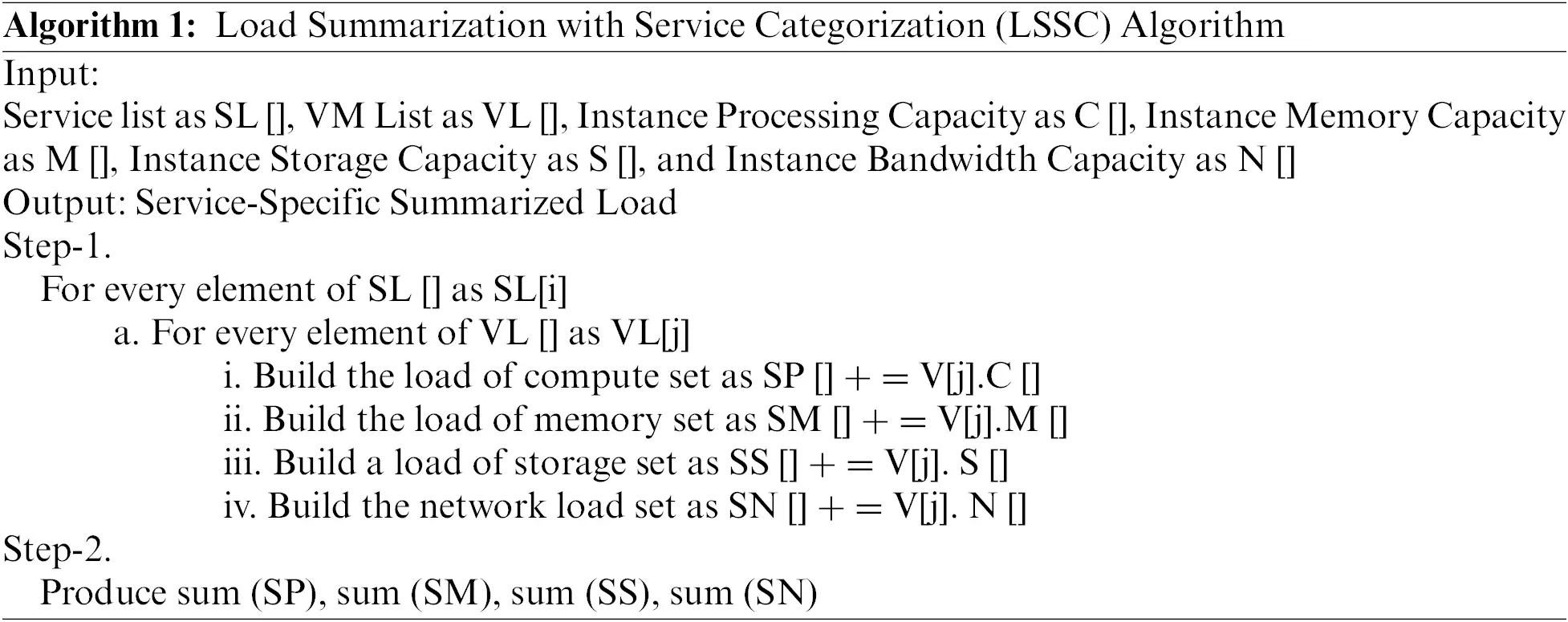Secondly, the pheromone-level prediction algorithm is furnished.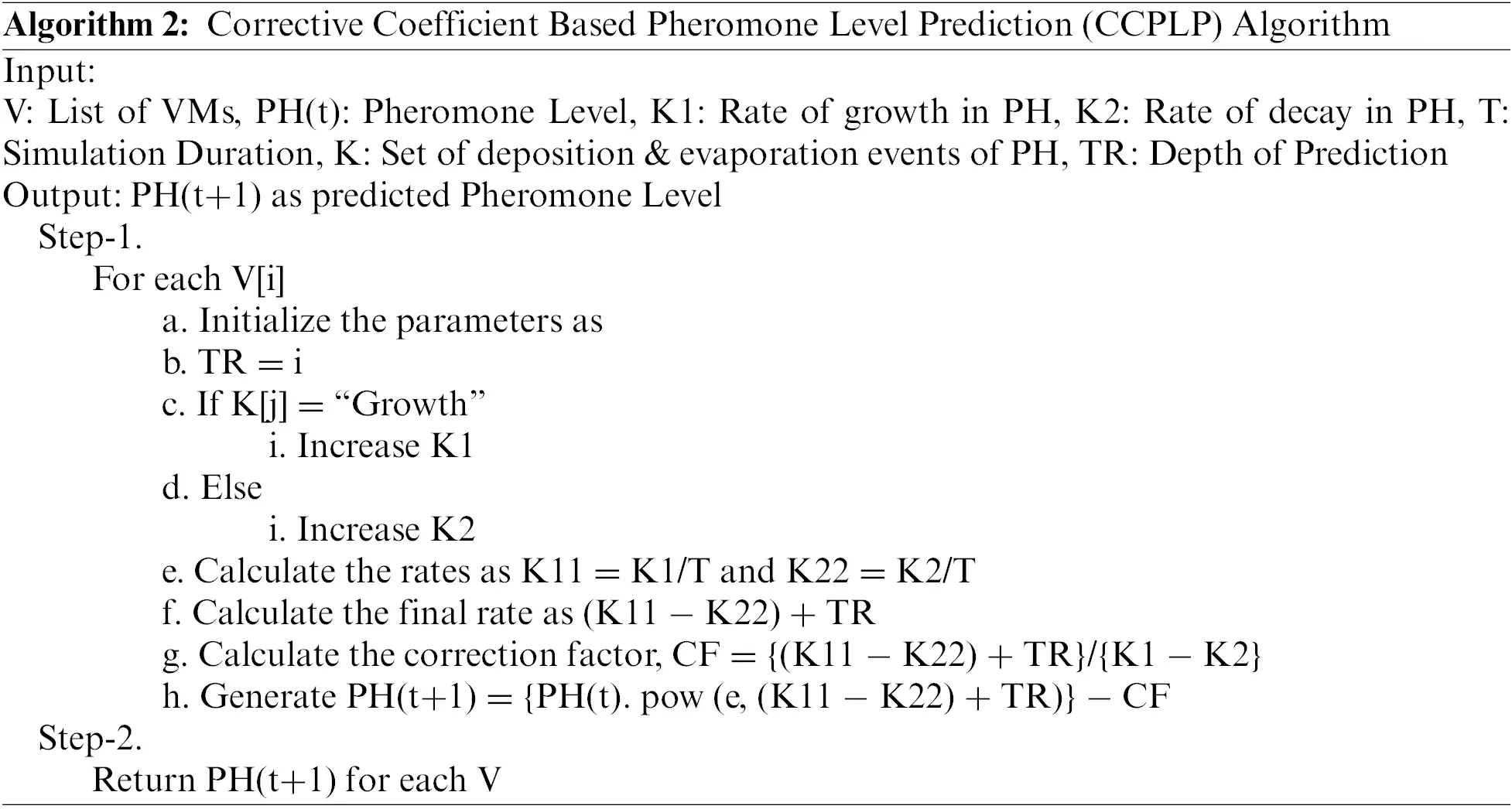To better understand the advantages of the Algorithm, examples of real-world data are used. Analytical input is referred to as “input data,” as shown in Table 1. Own Data from practical experimentation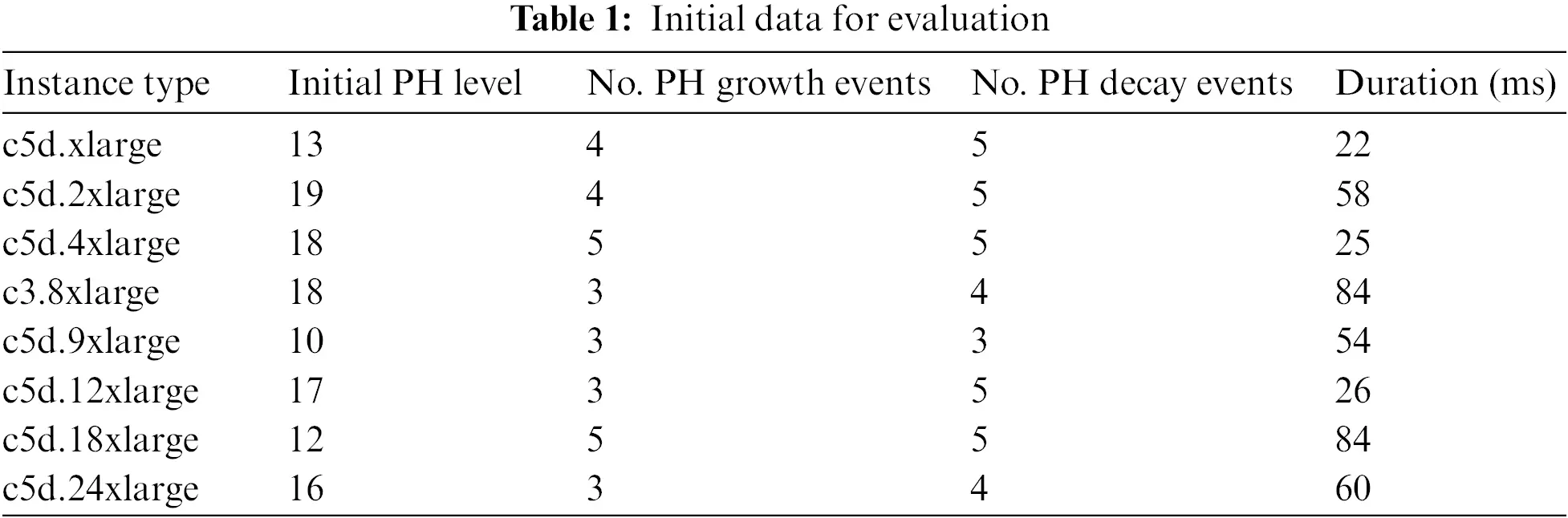After the Initial PH level and the growth or decay events are identified, in the next phase, the growth rate and the decay rate are calculated as shown in Table 2.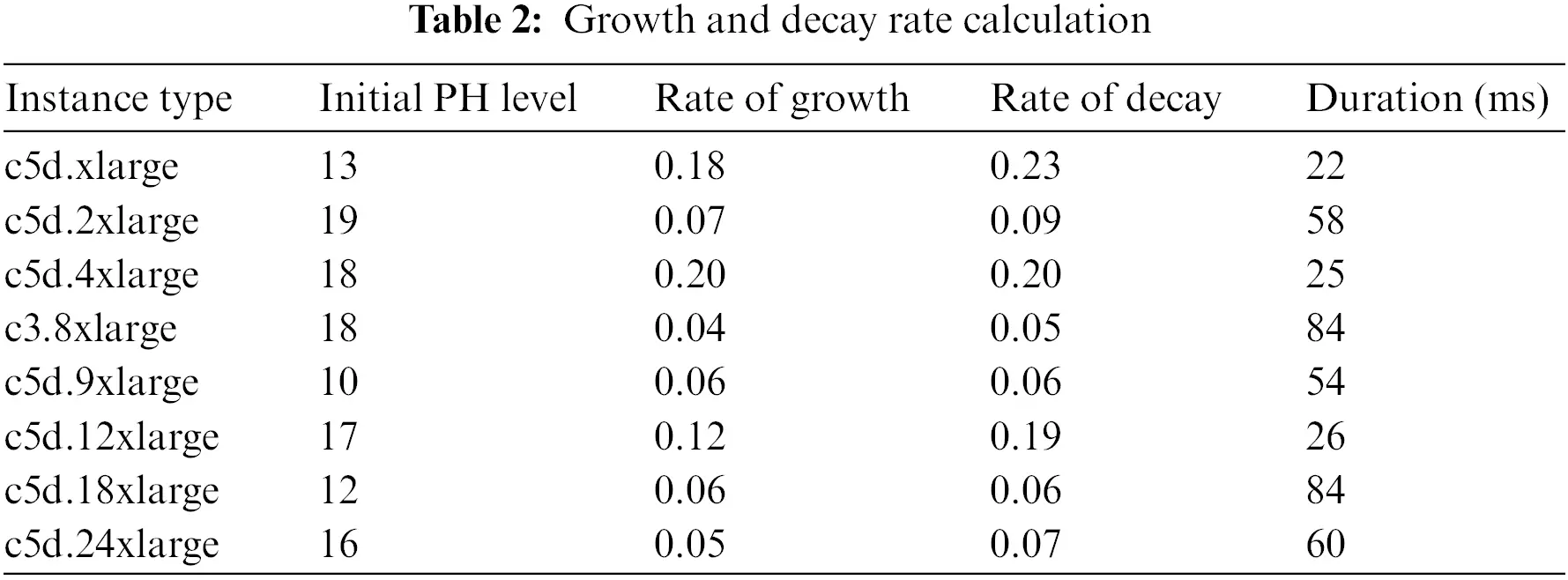Further, based on the mentioned steps in the Algorithm, the predictive value for the PH levels is compared with the actual PH level values. In this process, the correction factors are also calculated, as shown in Table 3.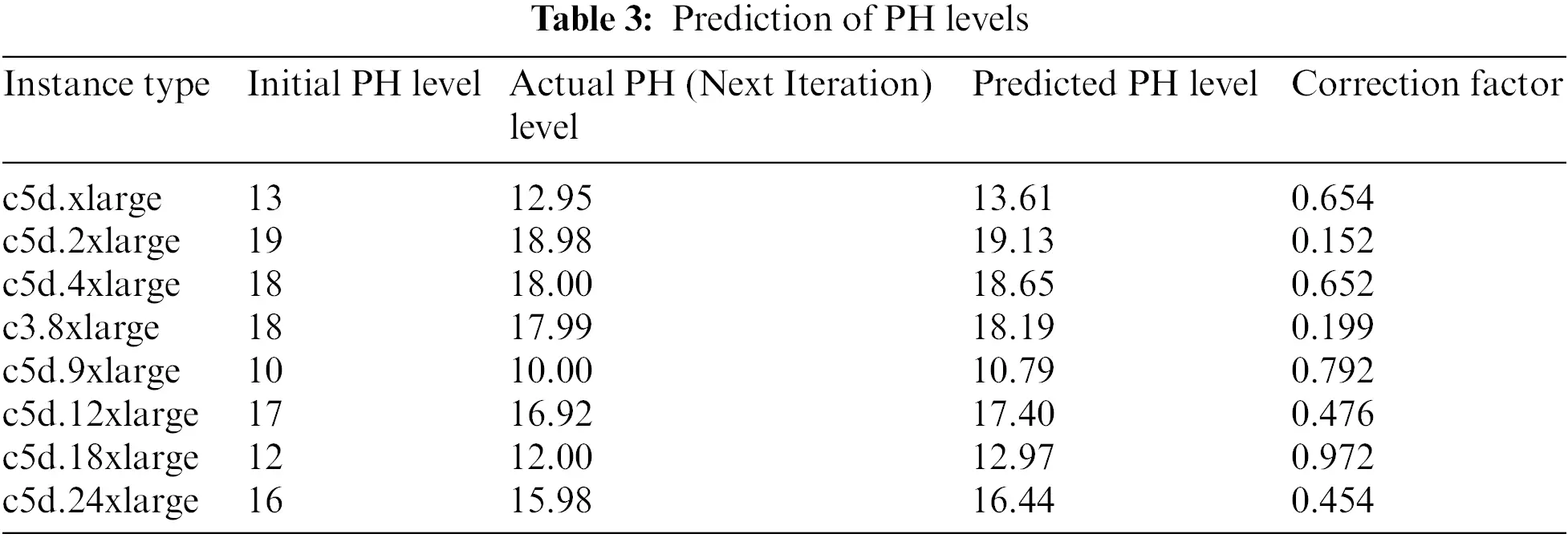As this Algorithm is an iterative process, the correction factors shall become zero in further iterations, and the actual PH level shall equate with the predicted PH levels. Thirdly, the load prediction algorithm is elaborated.The benefits of the Algorithm are better understood with the help of sample data. As a starting point, this data is referred to as Table 4.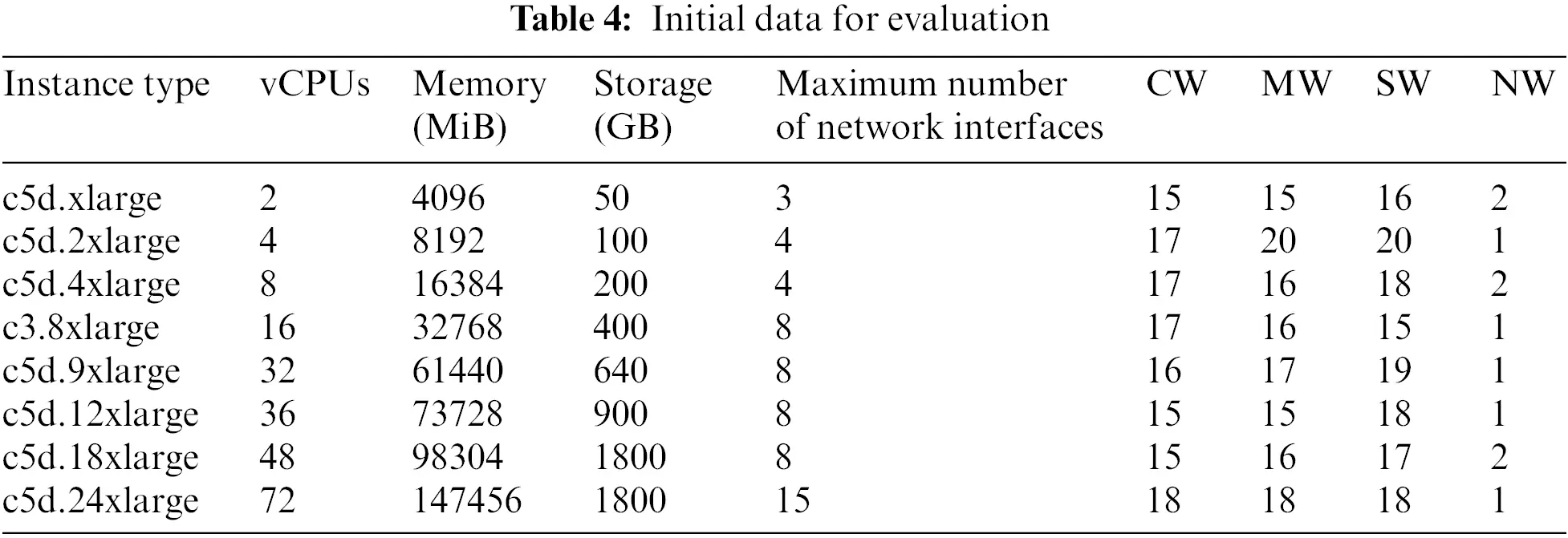The parallel research results recommend deriving the weight constants from the source course. For this location, and under the assumption that the c5d.xlarge available physical pool is overburdened, a load prediction is performed, as shown in Table 5.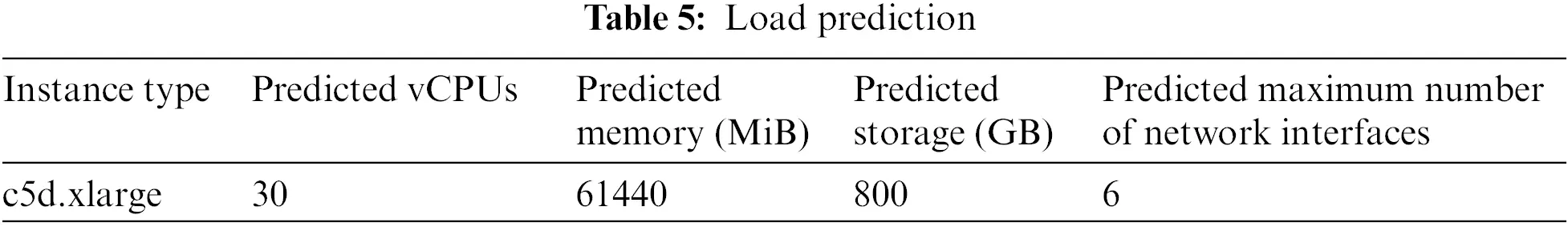The strength calculations are performed in the next step of the Algorithm. Over or justified capacity is shown by the positive numbers, while under capacity is shown by the negative numbers in Table 6.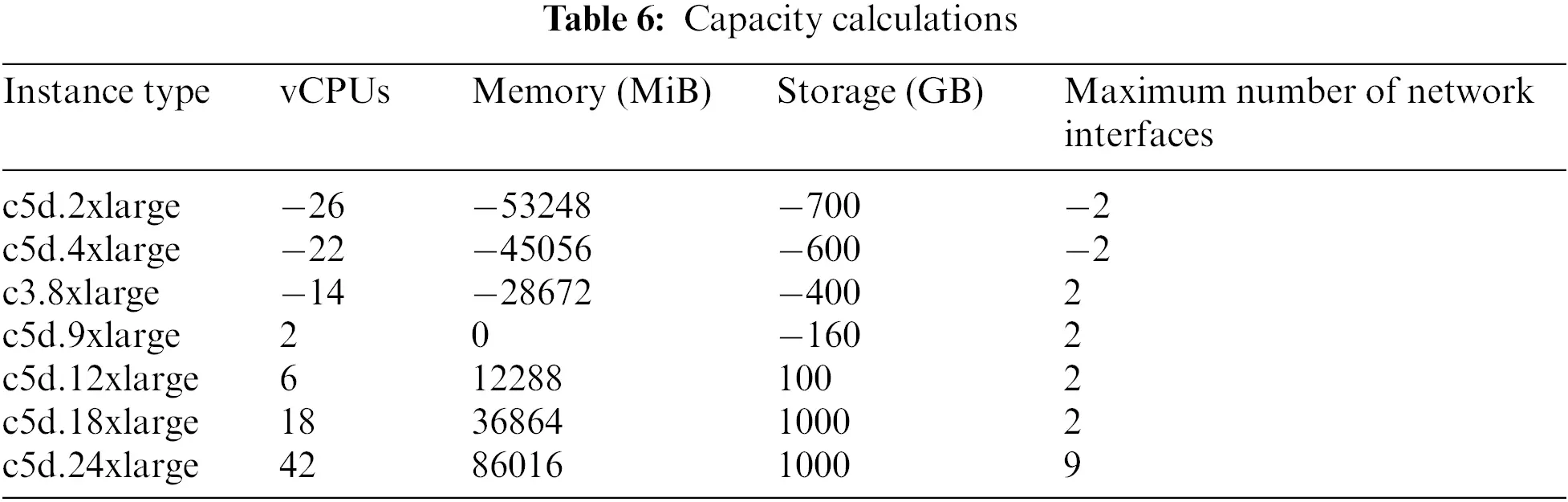As a result, c5d.12xlarge, c5d.18xlarge, and c5d.24xlarge are the migration options that can be considered. The live vm migration methodology for task scheduling is presented as a final example.

The benefits of the Algorithm are better understood with the help of sample data. As a starting point, this data is referred to in Table 7.Based on the capacity, it is natural to realize that c5d.9xlarge, c5d.12xlarge, c5d.18xlarge, and c5d.24xlarge are the feasible migration options. Thus, based on the proposed optimization fitness function, the capacity must be optimal, and the pheromone level must be highest. Hence, c5d.9xlarge is selected for the migration destination. Hence, based on the complete analysis, it is observed that the most cost-effective physical resource pool is selected for the migration. The more relevant proof is generated in the next Section of this work.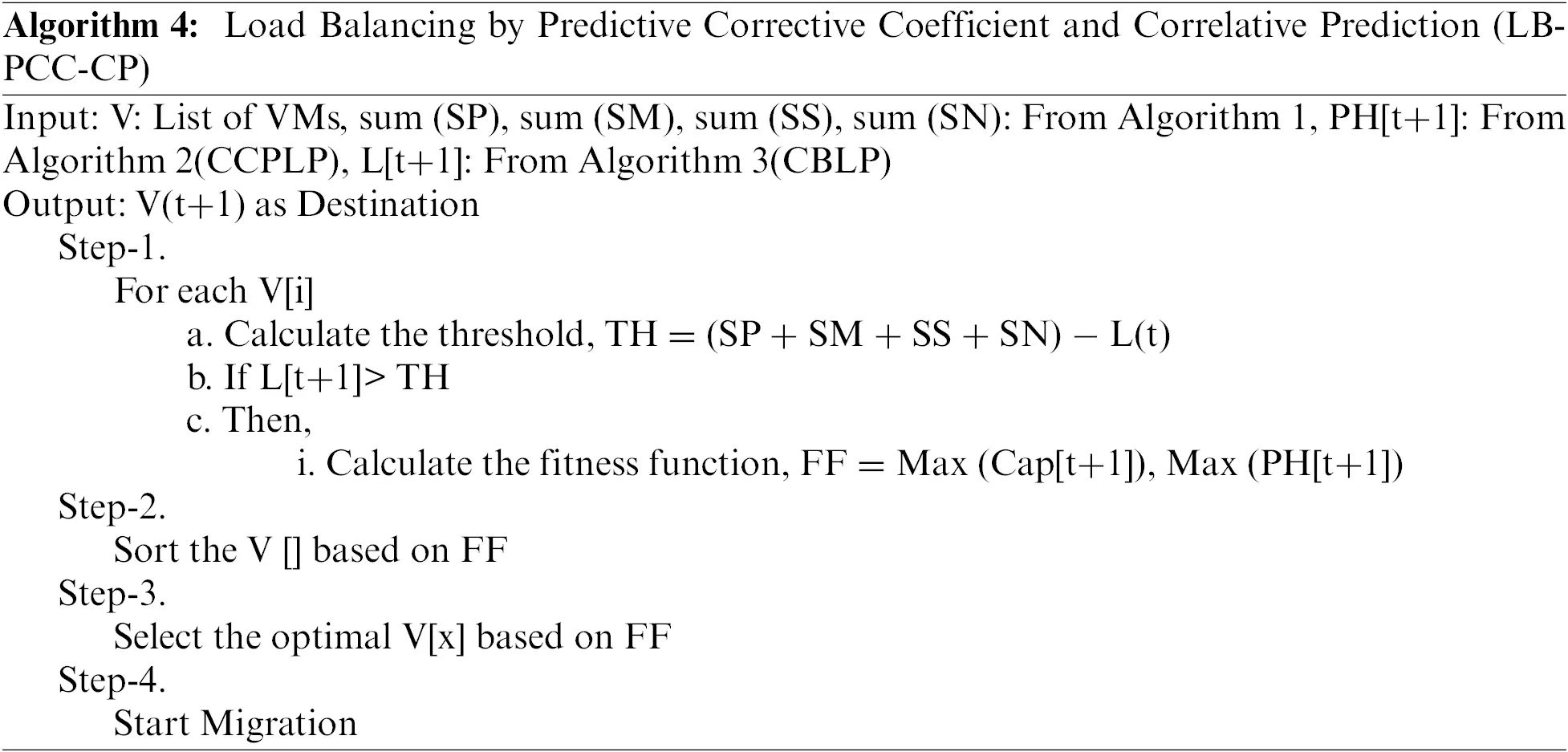:

Fig. 1 shows the visualization of the continuous improvements.Figure 1: Visualization of the continuous improvements

Henceforth, after the detailed discussion of the proposed Algorithm, in the next Section of the work, the results are analyzed.

4  Comparative Analysis

After understanding the load summarization and balancing process, primitive and recent methods for optimizations with the fundamental bottlenecks, formulating the problems to be solved, the mathematical models for the proposed solutions, and proposed algorithms based on the mathematical models, in this Section of the work, the obtained results are furnished. The results are simulated in controlled hardware and tested on the amazon web service platform for better results. The simulation is carried out firstly on CloudSim and AWS EC2 Services. The results obtained from CloudSim are furnished here. During this simulation, the performance measure parameters such as Energy consumption, Number of VM migrations, Violation of SLA, Number of host shutdowns, and total Execution time are considered. The algorithms are simulated over 10 PlanetLab  datasets and compared with the primitive algorithms such as “iqr_mmt, iqr_mu, iqr_rs, lrr_mc, lrr_mmt, lrr_mu, lrr_rs, lr_mc, lr_mmt, lr_mu, lr_rs, mad_mc, mad_mmt, mad_mu, mad_rs, thr_mc, thr_mmt, thr_mu and thr_rs”. Finally, in this Section of the work, the proposed method is compared with the other virtual machine migration method with the average value for each parameter discussed. The analysis is furnished here in Table 8.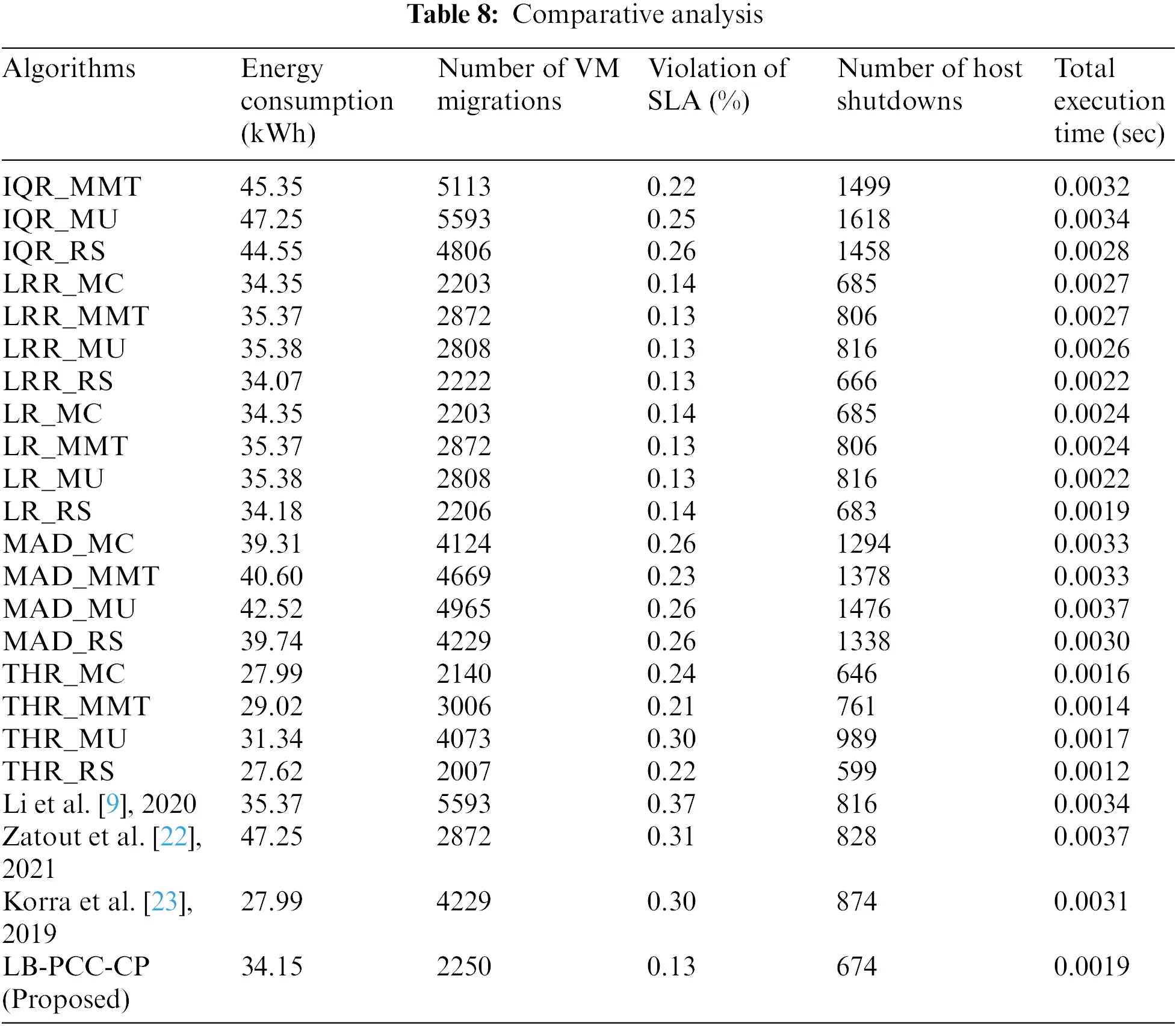From the analysis, it is observed that for Energy consumption as low as 34.15 (kWh) as better validation, the proposed method stands in 6th position. For the Number of VM migrations as low as 2250 as better validation, the proposed method stands at 7th position. For Violation of SLA as low as 0.13(%) as better validation, the proposed method stands at 1st position. For the Number of host shutdowns as low as 674 as better validation, the proposed method stands at 4th position. For total Execution time as low as 0.0019 (sec) as better validation, the proposed method stands at the 5th position, ensuring the performance in the upper half during the total analysis. Hence, this is conclusive that the proposed model is a highly stable performance-improved method for load balancing strategy.

5  Conclusion

The current load-balancing systems have nearly explored genetic optimization strategies. As a result, load-balancing solutions can only improve response times up to a degree. These optimization approaches are criticized for being less dynamic, rule-based, and less effective on virtualized resources. To optimize load balance, this study presents a unique approach for predictive load estimate, reduction, or summarization, combining correlation-based parametric reduction and correction coefficient-based pheromone prediction. This work shows the solution of long-standing problems using standard optimization methods, such as highly complex probability distributions, higher complexity of the solution search space for ACO using predictive analysis of the pheromone level, non-coordinated search space problem, which cannot be solved by PSO, and increasing complexity problem while in ACO. The findings show a considerable decrease in response time or time complexity for optimization resulting in fewer SLA violations and a significant reduction in virtual machine migrations compared to other standard benchmarked techniques. From the analysis, we can see that for Energy consumption as low as better validation, the proposed method ranks 6th. For the Number of VM migrations as low as better validation, it ranks 7th. For Violation of SLA as low as better validation, it ranks 1st. For the Number of host shutdowns as low as better validation, it ranks 4th, and for total Execution time as low as better validation, it ranks 1st. So, the proposed model is an improved load-balancing method that is stable and works well.

Acknowledgement: The authors would like to thank the R&D departments of K. L. University, Vaddeswaraam, and B. V. Raju Institute of Technology for supporting this work.

Funding Statement: The authors received no specific funding for this study.

Conflicts of Interest: The authors declare that they have no conflicts of interest to report regarding the present study.

## References

1. B. P. Rimal, E. Choi and I. Lumb, “A taxonomy and survey of cloud computing systems,” in 2009 Fifth Int. Joint Conf. on INC, IMS and IDC, Seoul, Korea (Southpp. 44–51, 2009.
2. S. Patidar, D. Rane and P. Jain, “A survey paper on cloud computing,” in 2012 Second Int. Conf. on Advanced Computing & Communication Technologies, Rohtak, India, pp. 394–398, 201
3. J. Kaur, M. Kaur and S. Vashist, “Virtual machine migration in cloud data centers,” International Journal of Advanced Research in Computer Science and Software Engineering, vol. 4, no. 8, pp. 190–193, 2014.
4. D. G. Chandra and D. B. Malaya, “A study on cloud os,” in Int. Conf. on Advanced Computing and Communication Technologies, Rajkot, Gujarat, India, pp. 692–697, 2012.
5. M. Mishra, A. Das, P. Kulkarni and A. Sahoo, “Dynamic resource management using virtual machine migrations,” IEEE Communications Magazine, vol. 50, no. 9, pp. 34–40, 2012.
6. A. Agarwal and S. Raina, “Live migration of virtual machines in the cloud,” International Journal of Scientific and Research Publication, vol. 2, pp. 1–5, 2012.
7. M. R. Barzoki and S. R. Hejazi, “Pseudo-polynomial dynamic programming for an integrated due date assignment, resource allocation, production, and distribution scheduling model in supply chain scheduling,” Applied Mathematical Modelling, vol. 39, no. 12, pp. 3280–3289, 2015.
8. M. Akbari and H. Rashidi, “A multi-objectives scheduling algorithm based on cuckoo optimization for task allocation problem at compile time in heterogeneous systems,” Expert Systems with Applications, vol. 60, pp. 234–248, 2016.
9. Y. Li, Y. Wen, D. Tao and K. Guan, “Transforming cooling optimization for green data center via deep reinforcement learning,” IEEE Transactions on Cybernetics, vol. 50, no. 5, pp. 2002–2013, 2020.
10. J. Zhou, Y. Zhang, L. Sun, S. Zhuang, C. Tang et al., “Stochastic virtual machine placement for cloud data centers under resource requirement variations,” IEEE Access, vol. 7, pp. 174412–174424, 2019.
11. S. Yan, Y. Zhang, S. Tao, X. Li and J. Sun, “A stochastic virtual machine placement algorithm for energy-efficient cyber-physical cloud systems,” in 2019 Int. Conf. on Internet of Things (iThings) and IEEE Green Computing and Communications (GreenCom) and IEEE Cyber, Physical and Social Computing (CPSCom) and IEEE Smart Data (SmartData), Atlanta, GA, USA, pp. 587–594, 2019.
12. V. Medina and J. M. García, “A survey of migration mechanisms of virtual machines,” ACM Computer Surveys, vol. 46, no. 3, pp. 1–30, 2014.
13. W. Wen, C. Wang, D. Wu and Y. Xie, “An act-based scheduling strategy on load balancing in a cloud computing environment,” in 2015 Ninth Int. Conf. on Frontier of Computer Science and Technology, Dalian, pp. 364–369, 2015.
14. X. Zhou, G. Zhang, J. Sun, J. Zhou, T. Wei et al., “Minimizing cost and makes pan for workflow scheduling in the cloud using fuzzy dominance sort based HEFT,” Future Generation Computer Systems, vol. 93, no. 5, pp. 278–289, 2019.
15. X. Zhang, T. Wu, M. Chen, T. Wei, J. Zhou et al., “Energy-aware virtual machine allocation for the cloud with resource reservation,” Journal of Systems and Software, vol. 147, no. 5, pp. 147–161, 2019.
16. V. Selvi and R. Umarani, “Comparative analysis of ant colony and particle swarm optimization techniques,” International Journal of Computer Applications, vol. 5, no. 4, 2010.
17. M. Juneja and S. K. Nagar, “Particle swarm optimization algorithm and its parameters: A review,” in 2016 Int. Conf. on Control, Computing, Communication and Materials (ICCCCM), Allahabad, pp. 1–5, 2016.
18. Y. Xu, P. Fan and L. Yuan, “A simple and efficient artificial bee colony algorithm,” Mathematical Problems in Engineering, vol. 2013, no. 14, pp. 1–9, 2013.
19. M. H. Kashan, N. Nahavandi and A. H. Kashan, “DisABC: A new artificial bee colony algorithm for binary optimization,” Applied Soft Computing, vol. 12, no. 1, pp. 342–352, 2012.
20. Y. Zhang and J. Sun, “Novel efficient particle swarm optimization algorithms for solving qos-demanded bag-of-tasks scheduling problems with profit maximization on hybrid clouds,” Concurrency Computation Practice Experience, vol. 29, no. 21, pp. e4249, 2017.
21. S. Sharma, D. S. Verma, D. K. Jyoti and D. Kavita, “Hybrid bat algorithm for balancing load in cloud computing,” International Journal of Engineering & Technology, vol. 7, no. 4, pp. 26, 2018.
22. M. S. Zatout, A. Rezoug, A. Rezoug and K. Baizid, “Optimization of fuzzy logic quadrotor attitude controller—particle swarm, cuckoo search and bat algorithms,” International Journal of Systems Science, vol. 52, no. 4, pp. 883–908, 2021.
23. S. Korra, D. Vasumathi and A. Vinayababu, “A framework for software component reusability analysis using flexible software components extraction,” International Journal of Recent Technology and Engineering, vol. 8, no. 2S3, pp. 1359–1367, 2019.
24. M. Labbadi, Y. Boukal and M. Cherkaoui, “Path following control of quadrotor uav with continuous fractional-order super twisting sliding mode,” Journal of Intelligent & Robotic Systems, vol. 100, no. 3, pp. 1429–1451, 2020.
25. M. K. A. Sharman, B. J. Emran, M. A. Jaradat, H. Najjaran, R. A. Husari et al., “Precision landing using an adaptive fuzzy multi-sensor data fusion architecture,” Applied Soft Computing, vol. 69, no. 4, pp. 149–164, 2018.
26. P. Kollapudi, S. Alghamdi, N. Veeraiah, Y. Alotaibi, S. Thotakura et al., “A new method for scene classification from the remote sensing images,” Computers, Materials & Continua, vol. 72, no. 1, pp. 1339–1355, 2022.
27. W. Jun and S. Xiaowei, “Research on sdn load balancing of ant colony optimization algorithm based on computer big data technology,” in 2022 IEEE Int. Conf. on Advances in Electrical Engineering and Computer Applications (AEECA), Dalian, China, pp. 935–938, 2022.
28. A. Yadav, S. J. Goyal, R. S. Jadon and R. Goyal, “Energy efficient load balancing algorithm through metahuristics approaches for cloud-computing-environment,” in 2022 Int. Mobile and Embedded Technology Conf. (MECON), Noida, India, pp. 130–135, 2022.
29. A. Kaushik and H. S. A. Raweshidy, “A hybrid latency- and power-aware approach for beyond fifth-generation internet-of-things edge systems,” IEEE Access, vol. 10, pp. 87974–87989, 2022.

N. Dey, T. Gunasekhar and K. Purnachand, "Aco-inspired load balancing strategy for cloud-based data centre with predictive machine learning approach," Computers, Materials & Continua, vol. 75, no.1, pp. 513–529, 2023.This work is licensed under a Creative Commons Attribution 4.0 International License , which permits unrestricted use, distribution, and reproduction in any medium, provided the original work is properly cited.

View

Like

## Related articles

• Jen-Yuan Yeh
• R. Sujatha, Jyotir Moy Chatterjee,...
• K. S. Bhuvaneshwari, J. Uma, K....
• R. Anand Babu, S. Kannan
• R. S. Latha, K. Venkatachalam,...